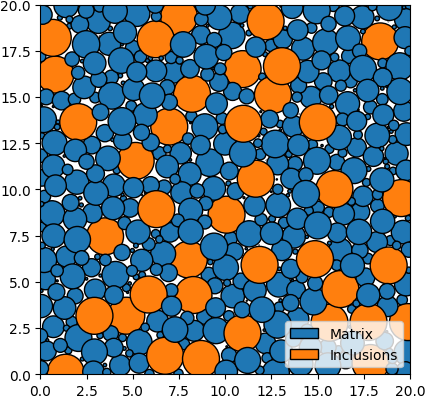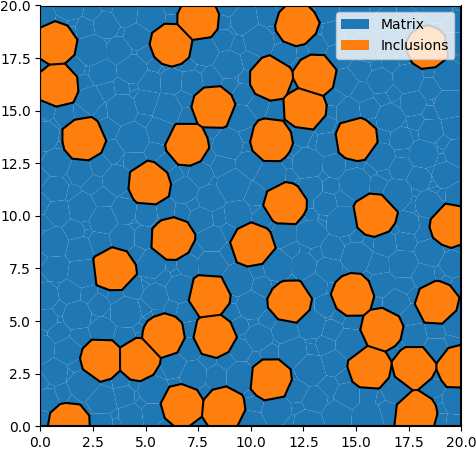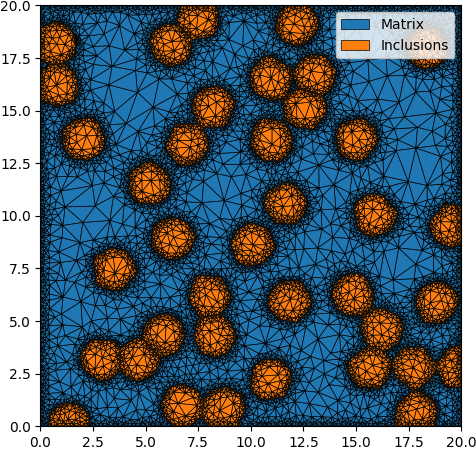# 2. Quality Controls¶

## 2.1. XML Input File¶

The basename for this file is `intro_2_quality.xml`. The file can be run using this command:

```microstructpy --demo=intro_2_quality.xml
```

The full text of the file is:

```<?xml version="1.0" encoding="UTF-8"?>
<input>
<material>
<name> Matrix </name>
<material_type> matrix </material_type>
<fraction> 2 </fraction>

<shape> circle </shape>
<size>
<dist_type> uniform </dist_type>
<loc> 0 </loc>
<scale> 1.5 </scale>
</size>
</material>

<material>
<name> Inclusions </name>
<fraction> 1 </fraction>
<shape> circle </shape>
<diameter> 2 </diameter>
</material>

<domain>
<shape> square </shape>
<side_length> 20 </side_length>
<corner> (0, 0) </corner>
</domain>

<settings>
<directory> intro_2_quality </directory>
<verbose> True </verbose>

<!-- Mesh Quality Settings -->
<mesh_min_angle> 25 </mesh_min_angle>
<mesh_max_volume> 1 </mesh_max_volume>
<mesh_max_edge_length> 0.1 </mesh_max_edge_length>
</settings>
</input>
```

## 2.2. Materials¶

There are two materials, in a 2:1 ratio based on volume. The first is a matrix, which is represented with small circles. The second material consists of circular inclusions with diameter 2.

## 2.3. Domain Geometry¶

These two materials fill a square domain. The bottom-left corner of the rectangle is the origin, which puts the rectangle in the first quadrant. The side length is 20, which is 10x the size of the inclusions to ensure that microstructure is statistically representative.

## 2.4. Settings¶

The first two settings determine the output directory and whether to run the program in verbose mode. The following settings determine the quality of the triangular mesh.

The minimum interior angle of the elements is 25 degrees, ensuring lower aspect ratios compared to the first example. The maximum area of the elements is also limited to 1, which populates the matrix with smaller elements. Finally, The maximum edge length of elements at interfaces is set to 0.1, which increasing the mesh density surrounding the inclusions and at the boundary of the domain.

Note that the edge length control is currently unavailable in 3D.

## 2.5. Output Files¶

The three plots that this file generates are the seeding, the polygon mesh, and the triangular mesh. These three plots are shown in Fig. 2.1 - Fig. 2.3.Fig. 2.1 Introduction 2 - seed geometries.¶Fig. 2.2 Introduction 2 - polygonal mesh.¶Fig. 2.3 Introduction 2 - triangular mesh.¶